js实现a*星寻路算法附源码下载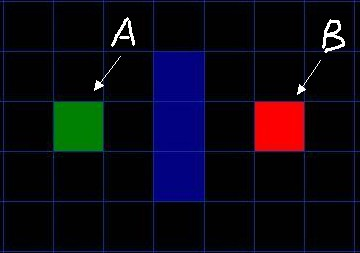1. 从起点A开始, 把它作为待处理的方格存入一个"开启列表", 开启列表就是一个等待检查方格的列表.

2. 寻找起点A周围可以到达的方格, 将它们放入"开启列表", 并设置它们的"父方格"为A.

3. 从"开启列表"中删除起点 A, 并将起点 A 加入"关闭列表", "关闭列表"中存放的都是不需要再次检查的方格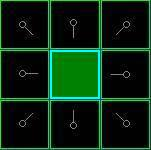F = G + H

G 表示从起点 A 移动到网格上指定方格的移动耗费 (可沿斜方向移动).

H 表示从指定的方格移动到终点 B 的预计耗费 (H 有很多计算方法, 这里我们设定只可以上下左右移动).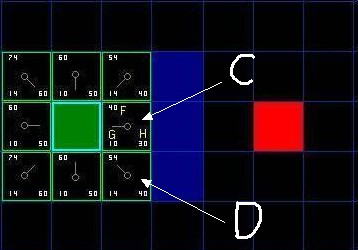4. 把它从 "开启列表" 中删除, 并放到 "关闭列表" 中.

5. 检查它所有相邻并且可以到达 (障碍物和 "关闭列表" 的方格都不考虑) 的方格. 如果这些方格还不在 "开启列表" 里的话, 将它们加入 "开启列表", 计算这些方格的 G, H 和 F 值各是多少, 并设置它们的 "父方格" 为 C.

6. 如果某个相邻方格 D 已经在 "开启列表" 里了, 检查如果用新的路径 (就是经过C 的路径) 到达它的话, G值是否会更低一些, 如果新的G值更低, 那就把它的 "父方格" 改为目前选中的方格 C, 然后重新计算它的 F 值和 G 值 (H 值不需要重新计算, 因为对于每个方块, H 值是不变的). 如果新的 G 值比较高, 就说明经过 C 再到达 D 不是一个明智的选择, 因为它需要更远的路, 这时我们什么也不做.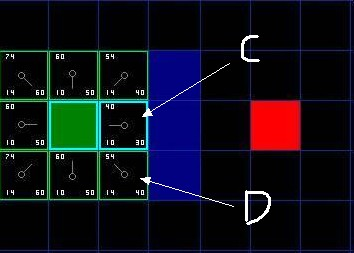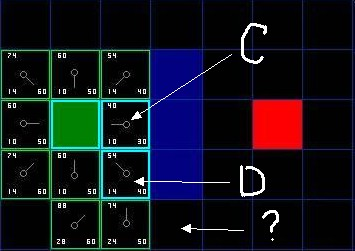D 右边已经右上方的都是墙, 所以不考虑, 但为什么右下角的没有被加进 "开启列表" 呢? 因为如果 C 下面的那块也不可以走, 想要到达 C 右下角的方块就需要从 "方块的角" 走了, 在程序中设置是否允许这样走. (图中的示例不允许这样走)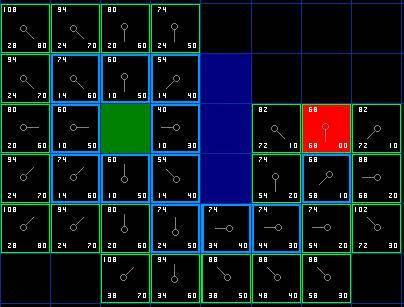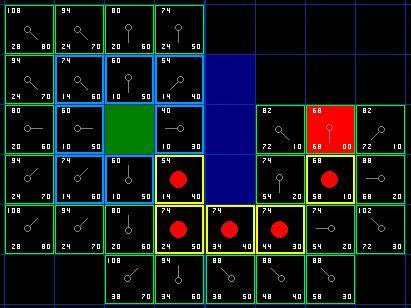do
{
寻找开启列表中F值最低的格子, 我们称它为当前格.
把它切换到关闭列表.
对当前格相邻的8格中的每一个
if (它不可通过 || 已经在 "关闭列表" 中)
{
什么也不做.
}
if (它不在开启列表中)
{
把它添加进 "开启列表", 把当前格作为这一格的父节点, 计算这一格的 FGH
if (它已经在开启列表中)
{
if (用G值为参考检查新的路径是否更好, 更低的G值意味着更好的路径)
{
把这一格的父节点改成当前格, 并且重新计算这一格的 GF 值.
}
} while( 目标格已经在 "开启列表", 这时候路径被找到)

js代码：

//其中的MAP.arr是二维数组
var openList=[],    //开启列表
closeList=[],   //关闭列表
result=[],      //结果数组
result_index;   //结果数组在开启列表中的序号

openList.push({x:start_x,y:start_y,G:0});//把当前点加入到开启列表中，并且G是0

do{
var currentPoint = openList.pop();
closeList.push(currentPoint);
var surroundPoint=SurroundPoint(currentPoint);
for(var i in surroundPoint) {
var item = surroundPoint[i];                //获得周围的八个点
if (
item.x>=0 &&                            //判断是否在地图上
item.y>=0 &&
item.x<MAP.rows &&
item.y<MAP.cols &&
MAP.arr[item.x][item.y] != 1 &&         //判断是否是障碍物
!existList(item, closeList) &&          //判断是否在关闭列表中
MAP.arr[item.x][currentPoint.y]!=1 &&   //判断之间是否有障碍物，如果有障碍物是过不去的
MAP.arr[currentPoint.x][item.y]!=1) {
//g 到父节点的位置
//如果是上下左右位置的则g等于10，斜对角的就是14
var g = currentPoint.G + ((currentPoint.x - item.x) * (currentPoint.y - item.y) == 0 ? 10 : 14);
if (!existList(item, openList)) {       //如果不在开启列表中
//计算H，通过水平和垂直距离进行确定
item['H'] = Math.abs(end_x - item.x) * 10 + Math.abs(end_y - item.y) * 10;
item['G'] = g;
item['F'] = item.H + item.G;
item['parent'] = currentPoint;
openList.push(item);
}
else {                                  //存在在开启列表中，比较目前的g值和之前的g的大小
var index = existList(item, openList);
//如果当前点的g更小
if (g < openList[index].G) {
openList[index].parent = currentPoint;
openList[index].G = g;
openList[index].F=g+openList[index].H;
}

}
}
}
//如果开启列表空了，没有通路，结果为空
if(openList.length==0) {
break;
}
openList.sort(sortF);//这一步是为了循环回去的时候，找出 F 值最小的, 将它从 "开启列表" 中移掉
}while(!(result_index=existList({x:end_x,y:end_y},openList)));

//判断结果列表是否为空
if(!result_index) {
result=[];
}
else {
var currentObj=openList[result_index];
do{
//把路劲节点添加到result当中
result.unshift({
x:currentObj.x,
y:currentObj.y
});
currentObj=currentObj.parent;
}while (currentObj.x!=start_x || currentObj.y!=start_y);

}
return result;

}
//用F值对数组排序
function sortF(a,b){
return b.F- a.F;
}
//获取周围八个点的值
function SurroundPoint(curPoint){
var x=curPoint.x,y=curPoint.y;
return [
{x:x-1,y:y-1},
{x:x,y:y-1},
{x:x+1,y:y-1},
{x:x+1,y:y},
{x:x+1,y:y+1},
{x:x,y:y+1},
{x:x-1,y:y+1},
{x:x-1,y:y}
]
}
//判断点是否存在在列表中，是的话返回的是序列号
function existList(point,list) {
for(var i in list) {
if(point.x==list[i].x && point.y==list[i].y) {
return i;
}
}
return false;
}

a*星寻路算法源码下载

HTML5基础入门视频教程，教学思路清晰，简单易学必会。适合人群：创业者，只要会打字，对互联网编程感兴趣都可以学。课程概述：该课程主要讲解HTML(学习HTML5的必备基础语言)、CSS3、Javascript(学习...
jquery视频教程从入门到精通，课程主要包含：jquery选择器、jquery事件、jquery文档操作、动画、Ajax、jquery插件的制作、jquery下拉无限加载插件的制作等等......
React是前端最火的框架之一，就业薪资很高，本课程教您如何快速学会React并应用到实战，对正在工作当中或打算学习React高薪就业的你来说，那么这门课程便是你手中的葵花宝典。

React是前端最火的框架之一，就业薪资很高，本课程教您如何快速学会React并应用到实战，对正在工作当中或打算学习React高薪就业的你来说，那么这门课程便是你手中的葵花宝典。